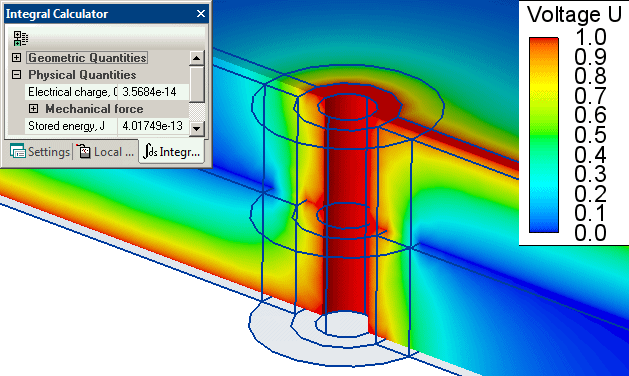QuickField

A new approach to field modelling
 Language: Global English Deutch Espanol Francais Italiano Danmark Ceske Chinese Pycckuü

Main >> Applications >> Sample problems >> PCB via capacitance

# PCB via capacitance

Problem Type: electrostatics.

Geometry: 3D extrusion
All dimensions are in millimetersGiven:
Relative permittivity of dielectric (FR-4) ε = 4.7;
Relative permittivity of air ε = 1.
Strip conductor potential V = 1 V.

Calculate the printed circuit board via capacitance.

Solution:
Capacitance can be calculated as C = 2·W / V2, where W is the electric field energy, V - potential difference.
In 3D problem there is no way to separate the strip electric field from the via electric field. Therefore the total energy is calculated and the resulting capacitance is of the via + strip together.

The 3D problem could be reduced to two 2D problems to calculate the via capacitance and strip conductor capacitance separately.

Results:
Electric potential distribution in the dielectric board (click to view 3D picture):Electric field energy (3D problem) W = 4.0e-13 J
Via and strip combined capacitance
C = 2*4.0e-13 / 12 = 8e-13 F (0.80 pF).

Via and strip capacitance calculated as a sum of two values form 2D simulations is 0.77 pF, including:

• Via capacitance is 0.33 pF• Strip capacitance (strip total length is 8.72 mm) is 0.44 pF• View simulation report in PDF
• Download simulation files (files may be viewed using any QuickField Edition)..